Introduction to cvms

2019-09-29

Abstract

This vignette is an introduction to the package cvms.
Cross-Validation for Model Selection.

Contact author at r-pkgs@ludvigolsen.dk

Main functions

The main functions of cvms are:

• cross_validate()
• cross_validate_fn()
• validate()
• evaluate()
• baseline()
• combine_predictors()
• cv_plot()
• select_metrics()
• reconstruct_formulas()

The difference between cross_validate() and cross_validate_fn()

Originally, cvms only provided the option to cross-validate Gaussian and binomial regression models, fitting the models internally with the lm(), lmer(), glm(), and glmer() functions. The cross_validate() function has thus been designed specifically to work with those functions.

To allow cross-validation of custom model functions like support-vector machines, neural networks, etc., the cross_validate_fn() function has been added. You provide a model function and (if defaults fail) a predict function, and it does the rest (see examples below).

Examples

The dataset participant.scores comes with cvms.

Fold data

Create a grouping factor for subsetting of folds using groupdata2::fold(). Order the dataset by the folds.

participant age diagnosis score session .folds
9 34 0 33 1 1
9 34 0 53 2 1
9 34 0 66 3 1
8 21 1 16 1 1
8 21 1 32 2 1
8 21 1 44 3 1
2 23 0 24 1 2
2 23 0 40 2 2
2 23 0 67 3 2
1 20 1 10 1 2
1 20 1 24 2 2
1 20 1 45 3 2
6 31 1 14 1 2
6 31 1 25 2 2
6 31 1 30 3 2

Cross-validate a single model

Gaussian

RMSE MAE r2m r2c AIC AICc BIC Dependent Fixed
16.35261 13.75772 0.270991 0.270991 194.6218 195.9276 197.9556 score diagnosis
Fold Column Fold Target Prediction
.folds 1 33 51.00000
.folds 1 53 51.00000
.folds 1 66 51.00000
.folds 1 16 30.66667
.folds 1 32 30.66667
.folds 1 44 30.66667
Fold Column Fold RMSE MAE r2m r2c AIC AICc BIC
.folds 1 12.56760 10.72222 0.2439198 0.2439198 209.9622 211.1622 213.4963
.folds 2 16.60767 14.77778 0.2525524 0.2525524 182.8739 184.2857 186.0075
.folds 3 15.97355 12.87037 0.2306104 0.2306104 207.9074 209.1074 211.4416
.folds 4 20.26162 16.66049 0.3568816 0.3568816 177.7436 179.1554 180.8772
term estimate std.error statistic p.value Fold Fold Column
(Intercept) 51.00000 5.901264 8.642216 0.0000000 1 .folds
diagnosis -20.33333 7.464574 -2.723978 0.0123925 1 .folds
(Intercept) 53.33333 5.718886 9.325826 0.0000000 2 .folds
diagnosis -19.66667 7.565375 -2.599563 0.0176016 2 .folds
(Intercept) 49.77778 5.653977 8.804030 0.0000000 3 .folds
diagnosis -18.77778 7.151778 -2.625610 0.0154426 3 .folds
(Intercept) 49.55556 5.061304 9.791065 0.0000000 4 .folds
diagnosis -22.30556 6.695476 -3.331437 0.0035077 4 .folds
Folds Fold Columns Convergence Warnings Singular Fit Messages Other Warnings Warnings and Messages Family
4 1 0 0 0 list(Fold Column = character(0), Fold = integer(0), Type = character(0), Message = character(0)) gaussian

Binomial

Balanced Accuracy F1 Sensitivity Specificity Pos Pred Value Neg Pred Value AUC Lower CI Upper CI
0.7361111 0.8205128 0.8888889 0.5833333 0.7619048 0.7777778 0.7685185 0.5962701 0.9407669
Kappa MCC Detection Rate Detection Prevalence Prevalence
0.4927536 0.5048268 0.5333333 0.7 0.6
Sensitivities Specificities
1.0000000 0.0000000
1.0000000 0.0833333
0.9444444 0.0833333
0.9444444 0.1666667
0.9444444 0.2500000
0.8888889 0.2500000
Fold Column Prediction Target Pos_0 Pos_1 N
.folds 0 0 TP TN 7
.folds 1 0 FN FP 5
.folds 0 1 FP FN 2
.folds 1 1 TN TP 16

Repeated cross-validation

Let’s first add some extra fold columns. We will use the num_fold_cols argument to add 3 unique fold columns. We tell fold() to keep the existing fold column and simply add three extra columns. We could also choose to remove the existing fold column, if for instance we were changing the number of folds (k). Note, that the original fold column will be renamed to “.folds_1”.

participant age diagnosis score session .folds_1 .folds_2 .folds_3 .folds_4
10 32 0 29 1 4 4 3 1
10 32 0 55 2 4 4 3 1
10 32 0 81 3 4 4 3 1
2 23 0 24 1 2 3 1 2
2 23 0 40 2 2 3 1 2
2 23 0 67 3 2 3 1 2
4 21 0 35 1 3 2 4 4
4 21 0 50 2 3 2 4 4
4 21 0 78 3 3 2 4 4
9 34 0 33 1 1 1 2 3
Fold Column Balanced Accuracy F1 Sensitivity Specificity Pos Pred Value Neg Pred Value AUC
.folds_1 0.7361111 0.8205128 0.8888889 0.5833333 0.7619048 0.7777778 0.7685185
.folds_2 0.7361111 0.8205128 0.8888889 0.5833333 0.7619048 0.7777778 0.7777778
.folds_3 0.7083333 0.7894737 0.8333333 0.5833333 0.7500000 0.7000000 0.7476852
.folds_4 0.7361111 0.8205128 0.8888889 0.5833333 0.7619048 0.7777778 0.7662037

Cross-validating custom model functions

cross_validate_fn() works with regression (gaussian), binary classification (binomial), and multiclass classification (multinomial).

SVM

Let’s cross-validate a support-vector machine using the svm() function from the e1071 package. First, we will create a model function. You can do anything you want in it, as long as it takes the arguments train_data and formula and returns the fitted model object.

For the svm() function, the default predict function and settings within cross_validate_fn() works, so we don’t have to specify a predict function. In many cases, it’s probably safer to supply a predict function anyway, so you’re sure everything is correct. We will see how in the naive Bayes example below, but first, let’s cross-validate the model function. Note, that some of the arguments have changed names (models -> formulas, family -> type).

Naive Bayes

The naive Bayes classifier requires us to supply a predict function, so we will go through that next. First, let’s create the model function.

Now, we will create a predict function. This will usually wrap stats::predict() and just make sure, the predictions have the correct format. When type is binomial, the predictions should be a vector, or a one-column matrix / data frame, with the probabilities of the second class (alphabetically). That is, if we have the classes 0 and 1, it should be the probabilities of the observations being in class 1. The help file, ?cross_validate_fn, describes the formats for the other types (gaussian and multinomial).

The predict function should take the arguments test_data, model, and formula. You do not need to use the formula within your function.

With both functions specified, we are ready to cross-validate our naive Bayes classifier.

Evaluating predictions

Evaluate predictions from a model trained outside cvms. Works with regression (gaussian), binary classification (binomial), and multiclass classification (multinomial). The following is an example of multinomial evaluation.

Plot results

There are currently a small set of plots for quick visualization of the results. It is supposed to be easy to extract the needed information to create your own plots. If you lack access to any information or have other requests or ideas, feel free to open an issue.

Gaussian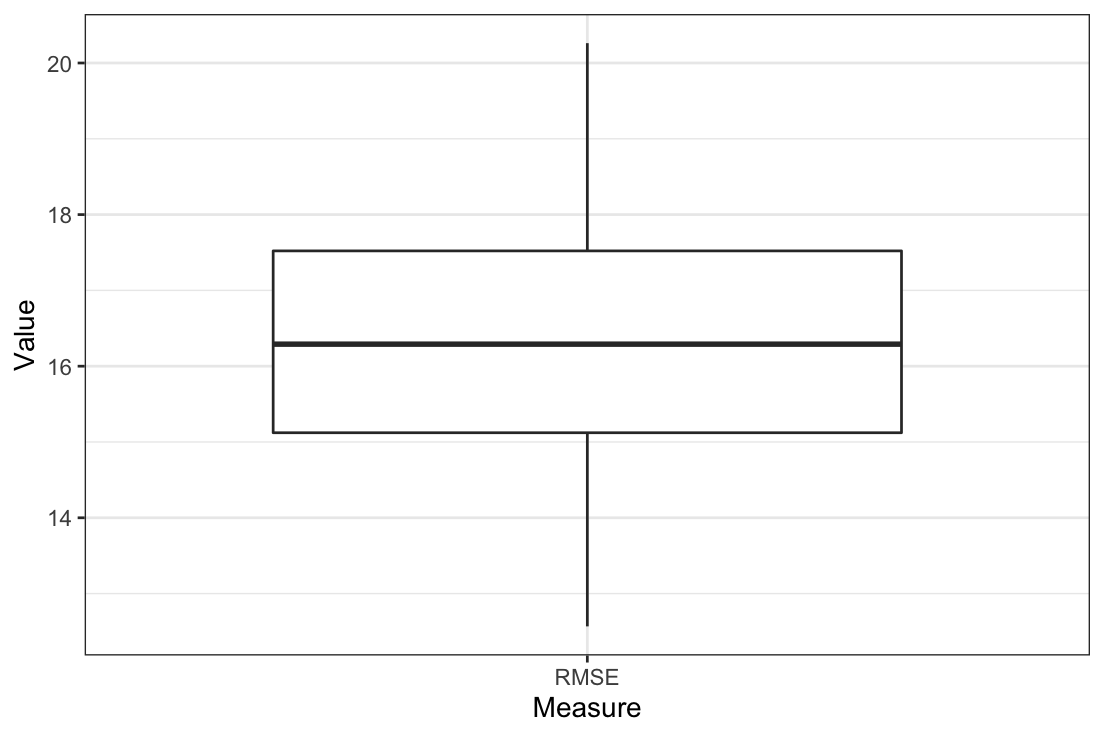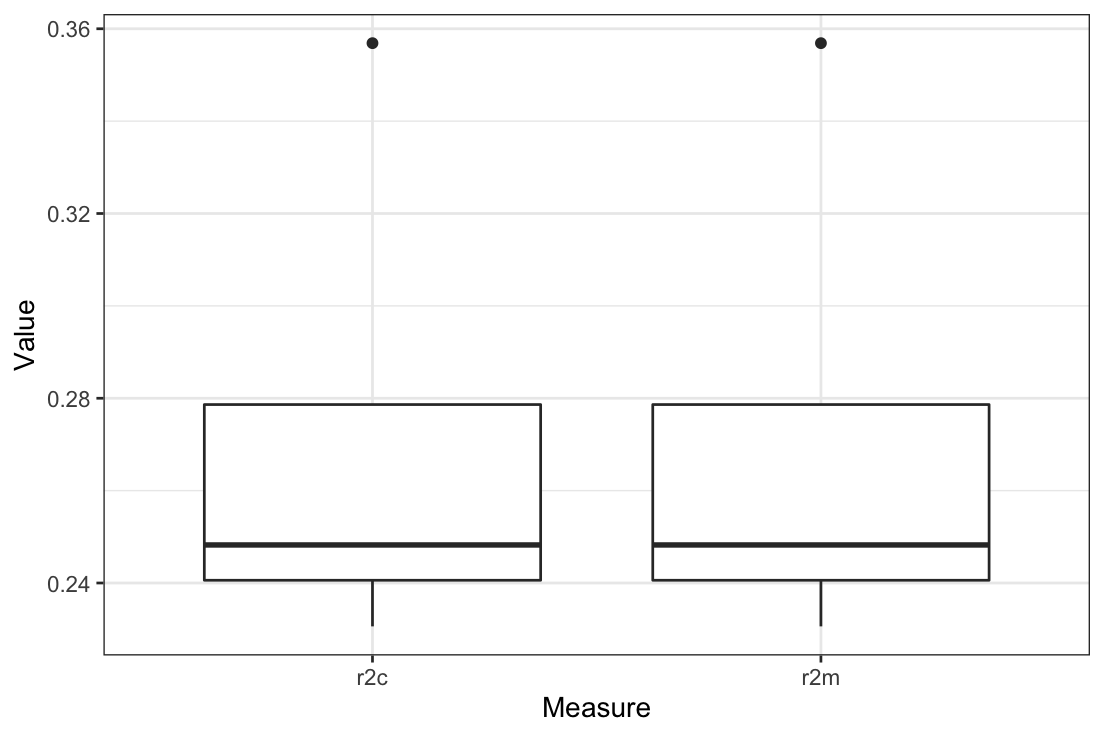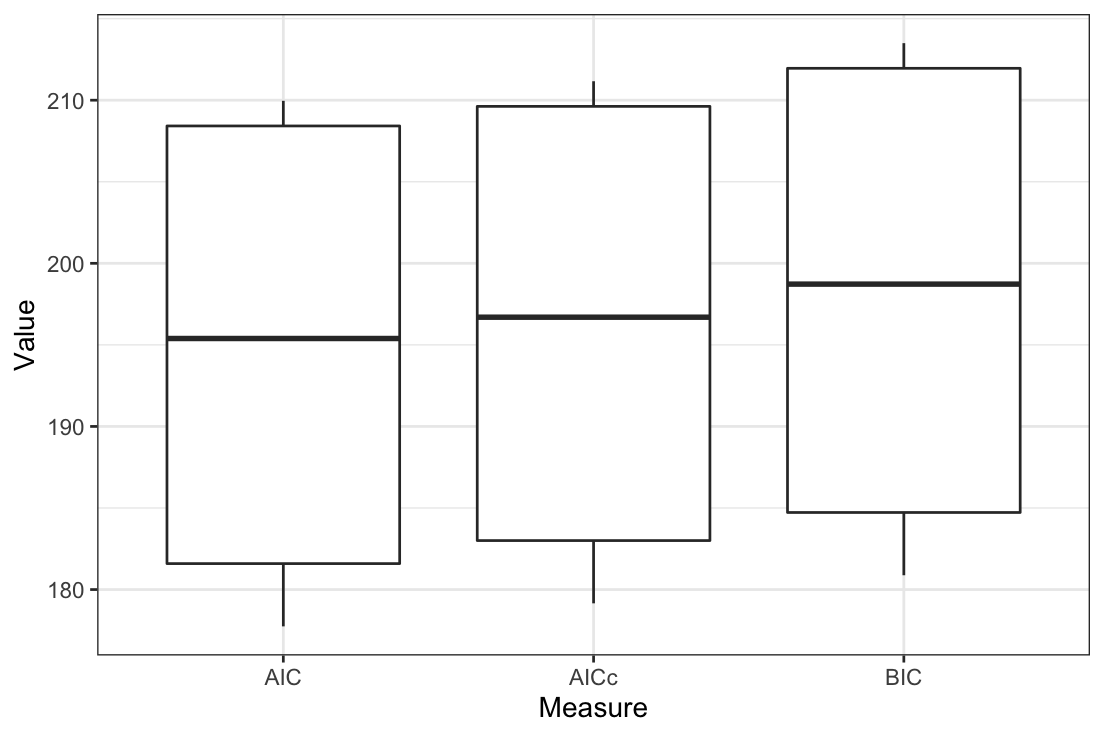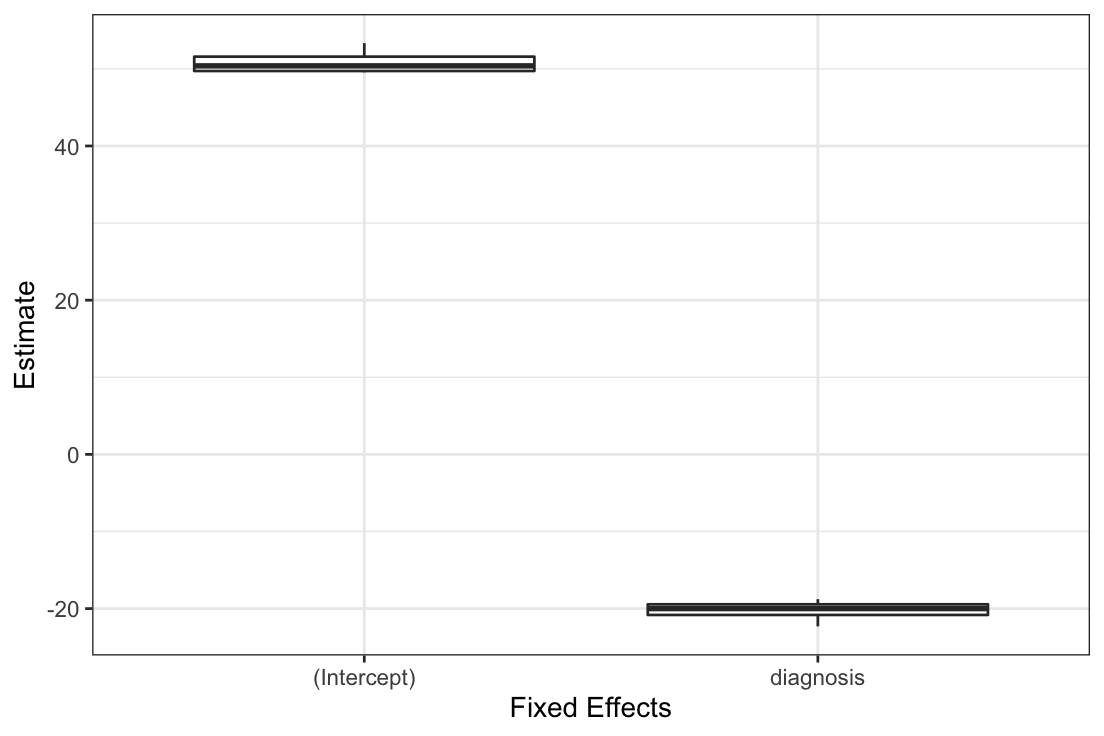Binomial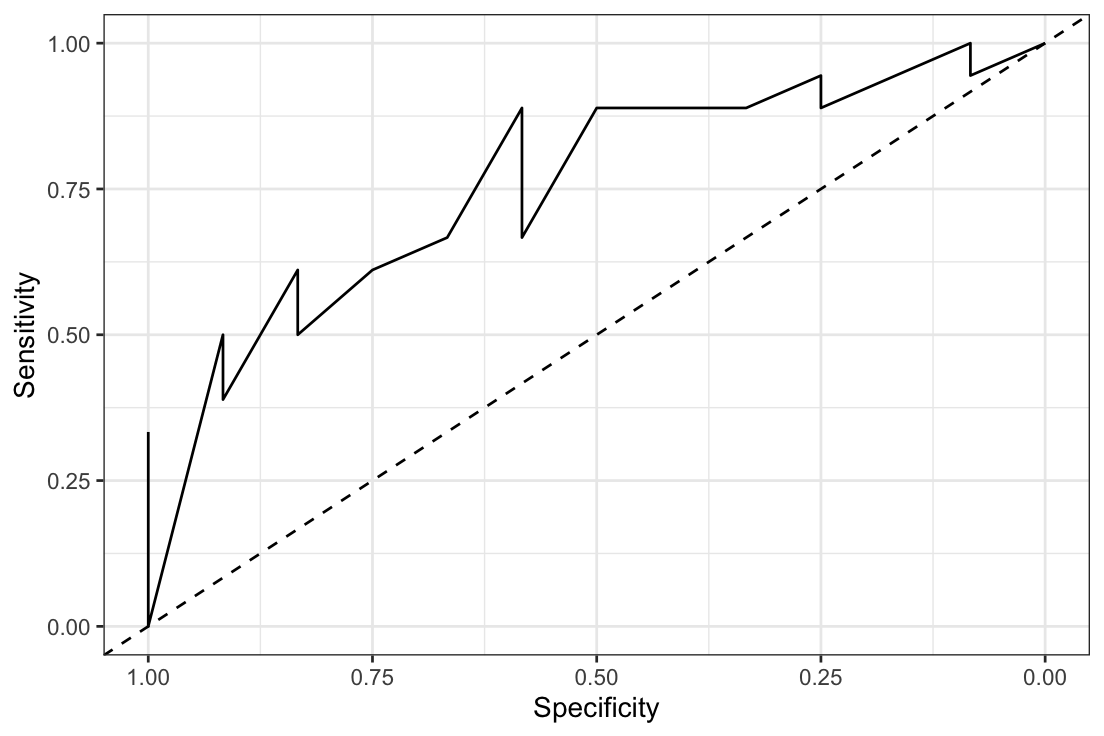Generate model formulas

Instead of manually typing all possible model formulas for a set of fixed effects (including the possible interactions), combine_predictors() can do it for you (with some constraints).

When including interactions, >200k formulas have been precomputed for up to 8 fixed effects, with a maximum interaction size of 3, and a maximum of 5 fixed effects per formula. It’s possible to further limit the generated formulas.

We can also append a random effects structure to the generated formulas.

If two or more fixed effects should not be in the same formula, like an effect and its log-transformed version, we can provide them as sublists.